#tianjara.net |Andrew Harvey's Blog

COMP3421 - Lec 1 - Colour
22nd August 2009

## Colour

Pure spectral light, is where the light source has just one single wavelength. This forms monochromatic (or pure spectral) colours.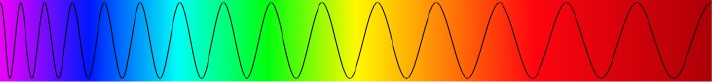However mostly light is made up of light of multiple wavelengths so you end up with a distribution of wavelengths. You could describe colour by this frequency distribution of wavelengths. For example brown is not in the spectrum, but we can get brown from this distribution of different light wavelengths,

[caption id="attachment_648" align="aligncenter" width="374" caption="Spectral Distribution for a Brown Colour (http://www.cs.rit.edu/~ncs/color/a_spectr.html)"]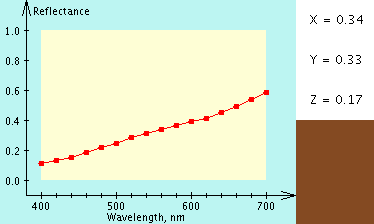[/caption]

We could describe colour like this (as opposed to RGB) but human eyes perceive many different distributions (spectral density functions) as the same colour (that is they are indistinguishable when placed side by side). The total power of the light is known as its luminance which is given by the area under the entire spectrum.

The human eye has three cones (these detect light), the short, medium and long cones (we have two kinds of receptors cones and rods, rods are good for detecting in low light but they cannot detect colour or fine detail). The graph below shows how these three cones respond to different wavelengths.

[caption id="attachment_649" align="aligncenter" width="300" caption="(Source: http://en.wikipedia.org/wiki/File:Cones_SMJ2_E.svg)"]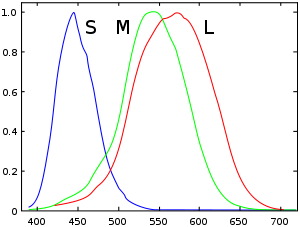[/caption]

So the colour we see is the result of our cones relative responses to RGB light. Because of this the human eye cannot distinguish some distributions that are different, to the eye they appear as the same color, hence you don't need to recreate the exact spectrum to create the same sensation of colour. We can just describe the colour as a mixture of three colours.

There are three CIE standard primaries X, Y, Z. An XYZ colour has a one to one match to RGB colour. (See http://www.cs.rit.edu/~ncs/color/t_spectr.html for the formulae.)

Not all visible colours can be produced using the RGB system.

=====

Where S, P, N are spectral functions,

if S = P then N + S = N + P (ie. we can add a colour to both sides and if they were perceived the same before, they will be percieved the same after)

On one side you project $S(\lambda)$ on the other you project combinations of A, B and C to give $aA(\lambda) + bB(\lambda) + cC(\lambda)$

By experimentation it was shown that to match any pure spectral colour $\lambda$ you needed the amounts of RGB shown,

=====

To detirmine the XYZ of a colour from its spectral distribution  you need to use the following equations,

$X= \int_0^\infty I(\lambda)\,\overline{x}(\lambda)\,d\lambda$ $Y= \int_0^\infty I(\lambda)\,\overline{y}(\lambda)\,d\lambda$ $Z= \int_0^\infty I(\lambda)\,\overline{z}(\lambda)\,d\lambda$

Where the $\overline{x}$, $\overline{y}$ and $\overline{z}$ functions are defined as,

[caption id="attachment_705" align="aligncenter" width="446" caption="The CIE 1931 XYZ colour matching functions. (Source: http://commons.wikimedia.org/wiki/File:CIE_1931_XYZ_Color_Matching_Functions.svg CC-BY-SA)"]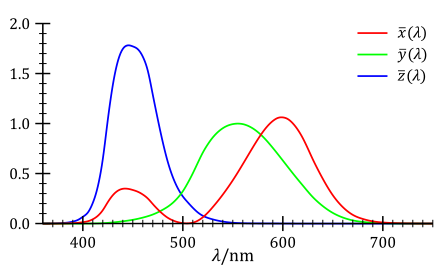[/caption]

## CIE Chromaticity Diagram

We can take a slice of the CIE space to get the CIE chromaticity diagram.

## RGB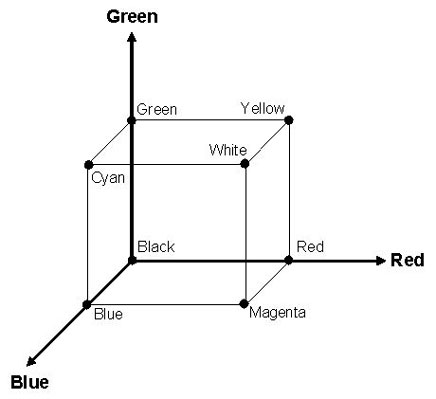(r, g, b) is the amount of red, green and blue primaries.

## CMY

CMY is a subtractive colour model (inks and paint works this way). (c,m,y) = (1,1,1) - (r,g,b).

But inks don't always subtract well so printers usually use a black ink as well using CMYK.

## HSV

The HSV colour model is really good for allowing the user to select a colour as they choose the hue (colour), saturation (how rich the colour is) and value (how dark the colour is).

[caption id="attachment_712" align="aligncenter" width="450" caption="HSV Colour Cone"]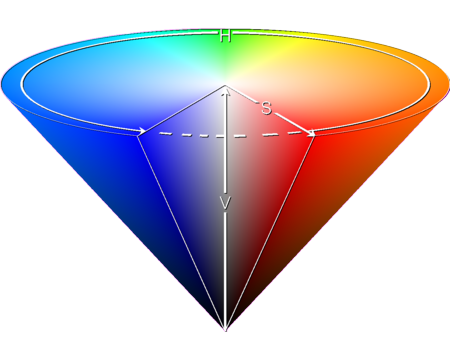[/caption]

## Gamut

Gamut is the range of colours available which is represented as a triangle in the CIE Chromacity diagram. Different devices have different gamuts (for instance the printer and LCD monitor).

• Gamut Clipping - A shading in one image becomes just a solid colour in the other.
• Gamut Scaling - Shading looks the same but the size of the gamut is minimal.

Tags: comp3421, graphics.